# Hands

The clock shows 12 hours. After how many minutes will angle between the hour and minute hand 90°?

Consider the continuous movement of both hands hours.

Result

x =  16.36 min

#### Solution:

360/60*x - 360/(12*60)*x = 90

330x = 5400

x = 18011 ≈ 16.363636

Calculated by our simple equation calculator.

Leave us a comment of this math problem and its solution (i.e. if it is still somewhat unclear...):Be the first to comment!#### Following knowledge from mathematics are needed to solve this word math problem:

Need help calculate sum, simplify or multiply fractions? Try our fraction calculator. Do you have a linear equation or system of equations and looking for its solution? Or do you have quadratic equation? Do you want to convert length units?

## Next similar math problems:

1. Forestry workersIn the forest is employed 56 laborers planting trees in nurseries. For 8 hour work day would end job in 37 days. After 16 days, 9 laborers go forth? How many days are needed to complete planting trees in nurseries by others, if they will work 10 hours a d
2. The machineThe machine works 7 hours a day and produces 1 part in 5 minutes. How many parts will it produce in 1 hour? How many parts will it produce in 1 day?
3. Two workers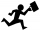One worker needs 40 hours to do a job, and the second would do it in 30 hours. They worked together for several hours, then the second was recalled, and the first completed the job itself in 5 hours. How many hours did they work together, and how much did.
4. Kilowatt-hours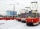If the Lewis family used 648 kilowatt-hours of electricity in 12 days, how many kilowatts hours should they use in 24 days at the same rate of usage
5. Video gameNicole is playing a video game where each round lasts 7/12 of an hour. She has scheduled 3 3/4 hours to play the game. How many rounds can Nicole play?
6. The touristThe tourist traveled 78km in 3 hours. Part of the journey went on foot at 6km/h, the rest of the trip by bus at 30km/h. How long did he walk?
7. PoolIf water flows into the pool by two inlets, fill the whole for 8 hours. The first inlet filled pool 6 hour longer than second. How long pool take to fill with two inlets separately?
8. Three cats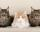If three cats eat three mice in three minutes, after which time 260 cats eat 260 mice?
9. Compound interest 3After 8 years, what is the total amount of a compound interest investment of \$25,000 at 3% interest, compounded quarterly? (interest is now dream - in the year 2019)
10. Car downSarah buys a car costing \$12,500. It depreciates in value by 8% in the first year, 10% the second year and 5% in the third year. Calculate the value of a car after the third year.
11. 45th birthdayThis year Mrs. Clever celebrated her 45th birthday. Her three children are now 7.11 and 15 years old. In how many years will Mrs. Clever's age be equal to the sum of her children's years?
12. Bike rideMarek rode a bike ride. In an hour, John followed him on the same route by car, at an average speed of 72 km/h, and in 20 minutes he drove him. Will he determine the length of the way that Marek took before John caught up with him, and at what speed did Ma
13. TroopsThe route is long 147 km and the first-day first regiment went at an average speed of 12 km/h and journey back 21 km/h. The second day went second regiment the same route at an average speed of 22 km/h there and back. Which regiment will take route longe
14. Propeller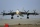The aircraft propeller rotates at an angular speed of 200 rad/s. A) What is the speed at the tip of the propeller if its distance from the axis of rotation is 1.5 m? B) What path does the aircraft travel during one revolution of the propeller at a speed
15. Reducing balance method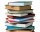A company buys an item having a useful life of 10 years for 1,000,000. If the company depreciates the item by the reducing balance method, a. Determine the depreciation for the first year. b. Estimate the depreciation for the second and third years. c..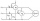Two wattmeters are connected to measuring power in a 3 phase balanced load. Determine the total power and power factor if the two wattmeters read 1000 watts each (1) both positive and (2) second reading is negativeThe sum of Robin's age is 45. Seven years ago, Robin was 16 years more than one half as old as Bruno then. How old is Bruno?